# Sphere

"Globose" redirects here. For the neuroanatomic structure, see Globose nucleus.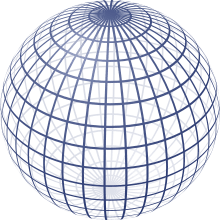A two-dimensional perspective projection of a sphere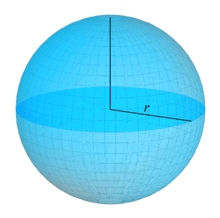r – radius of the sphere

A sphere (from Greek σφαῖραsphaira, "globe, ball") is a perfectly round geometrical object in three-dimensional space that is the surface of a completely round ball, (viz., analogous to a circular object in two dimensions).

Like a circle, which geometrically is a two-dimensional object, a sphere is defined mathematically as the set of points that are all at the same distance r from a given point, but in three-dimensional space. This distance r is the radius of the ball, and the given point is the center of the mathematical ball. The longest straight line through the ball, connecting two points of the sphere, passes through the center and its length is thus twice the radius; it is a diameter of the ball.

While outside mathematics the terms "sphere" and "ball" are sometimes used interchangeably, in mathematics a distinction is made between the sphere (a two-dimensional closed surface embedded in three-dimensional Euclidean space) and the ball (a three-dimensional shape that includes the sphere as well as everything inside the sphere). The ball and the sphere share the same radius, diameter, and center.

## Surface area

The surface area of a sphere is:Archimedes first derived this formula from the fact that the projection to the lateral surface of a circumscribed cylinder (for example, the Lambert cylindrical equal-area projection) is area-preserving; it equals the derivative of the formula for the volume with respect to r because the total volume inside a sphere of radius r can be thought of as the summation of the surface area of an infinite number of spherical shells of infinitesimal thickness concentrically stacked inside one another from radius 0 to radius r. At infinitesimal thickness the discrepancy between the inner and outer surface area of any given shell is infinitesimal, and the elemental volume at radius r is simply the product of the surface area at radius r and the infinitesimal thickness.

At any given radius r, the incremental volume (δV) equals the product of the surface area at radius r (A(r)) and the thickness of a shell (δr):The total volume is the summation of all shell volumes:In the limit as δr approaches zero this equation becomes:Substitute V:Differentiating both sides of this equation with respect to r yields A as a function of r:Which is generally abbreviated as:Alternatively, the area element on the sphere is given in spherical coordinates by dA = r2 sin θ dθ dφ. In Cartesian coordinates, the area element isFor more generality, see area element.

The total area can thus be obtained by integration:## Enclosed volume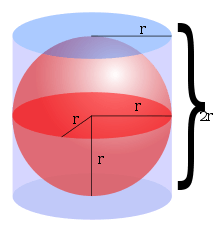Circumscribed cylinder to a sphere

In three dimensions, the volume inside a sphere (that is the volume of a ball) is derived to bewhere r is the radius of the sphere. Archimedes first derived this formula, which shows that the volume inside a sphere is 2/3 that of a circumscribed cylinder. (This assertion follows from Cavalieri's principle.) In modern mathematics, this formula can be derived using integral calculus, i.e. disk integration to sum the volumes of an infinite number of circular disks of infinitesimally small thickness stacked centered side by side along the x-axis from x = 0 where the disk has radius r (i.e. y = r) to x = r where the disk has radius 0 (i.e. y = 0).

At any given x, the incremental volume (δV) equals the product of the cross-sectional area of the disk at x and its thickness (δx):The total volume is the summation of all incremental volumes:In the limit as δx approaches zero this equation becomes:At any given x, a right-angled triangle connects x, y and r to the origin; hence, applying the Pythagorean theorem yields:Thus, substituting y with a function of x gives:Which can now be evaluated as follows:Therefore the volume of a sphere is:Alternatively this formula is found using spherical coordinates, with volume elementsoFor most practical purposes, the volume inside a sphere inscribed in a cube can be approximated as 52.4% of the volume of the cube, since π/6  0.5236. For example, a sphere with diameter 1 meter has 52.4% the volume of a cube with edge length 1 meter, or about 0.524 m3.

In higher dimensions, the analog of a sphere is called a hypersphere, which encloses an n-ball. General recursive and non-recursive formulas exist for the volume of an n-ball.

## Equations in three-dimensional space

In analytic geometry, a sphere with center (x0, y0, z0) and radius r is the locus of all points (x, y, z) such thatThe points on the sphere with radius r can be parameterized viaA sphere of any radius centered at zero is an integral surface of the following differential form:This equation reflects that position and velocity vectors of a point traveling on the sphere are always orthogonal to each other.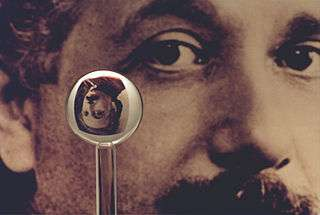An image of one of the most accurate human-made spheres, as it refracts the image of Einstein in the background. This sphere was a fused quartz gyroscope for the Gravity Probe B experiment, and differs in shape from a perfect sphere by no more than 40 atoms (less than 10 nanometers) of thickness. It was announced on 1 July 2008 that Australian scientists had created even more nearly perfect spheres, accurate to 0.3 nanometers, as part of an international hunt to find a new global standard kilogram.

The sphere has the smallest surface area of all surfaces that enclose a given volume, and it encloses the largest volume among all closed surfaces with a given surface area. The sphere therefore appears in nature: for example, bubbles and small water drops are roughly spherical because the surface tension locally minimizes surface area.

The surface area relative to the mass of a sphere is called the specific surface area and can be expressed from the above stated equations aswhere ρ is the density (the ratio of mass to volume).

A sphere can also be defined as the surface formed by rotating a circle about any diameter. Replacing the circle with an ellipse rotated about its major axis, the shape becomes a prolate spheroid; rotated about the minor axis, an oblate spheroid.

## Terminology

Pairs of points on a sphere that lie on a straight line through the sphere's center are called antipodal points. A great circle is a circle on the sphere that has the same center and radius as the sphere and consequently divides it into two equal parts. The shortest distance along the surface between two distinct non-antipodal points on the surface is on the unique great circle that includes the two points. Equipped with the great-circle distance, a great circle becomes the Riemannian circle.

If a particular point on a sphere is (arbitrarily) designated as its north pole, then the corresponding antipodal point is called the south pole, and the equator is the great circle that is equidistant to them. Great circles through the two poles are called lines (or meridians) of longitude, and the line connecting the two poles is called the axis of rotation. Circles on the sphere that are parallel to the equator are lines of latitude. This terminology is also used for such approximately spheroidal astronomical bodies as the planet Earth (see geoid).

## Hemisphere

Any plane that includes the center of a sphere divides it into two equal hemispheres. Any two intersecting planes that include the center of a sphere subdivide the sphere into four lunes or biangles, the vertices of which all coincide with the antipodal points lying on the line of intersection of the planes.

The antipodal quotient of the sphere is the surface called the real projective plane, which can also be thought of as the northern hemisphere with antipodal points of the equator identified.

The round hemisphere is conjectured to be the optimal (least area) filling of the Riemannian circle.

The circles of intersection of any plane not intersecting the sphere's center and the sphere's surface are called spheric sections.

## Generalizations

### Dimensionality

Main article: n-sphere

Spheres can be generalized to spaces of any number of dimensions. For any natural number n, an "n-sphere," often written as Sn, is the set of points in (n + 1)-dimensional Euclidean space that are at a fixed distance r from a central point of that space, where r is, as before, a positive real number. In particular:

• S0: a 0-sphere is a pair of endpoints of an interval [−r, r] of the real line
• S1: a 1-sphere is a circle of radius r
• S2: a 2-sphere is an ordinary sphere
• S3: a 3-sphere is a sphere in 4-dimensional Euclidean space.

Spheres for n > 2 are sometimes called hyperspheres.

The n-sphere of unit radius centered at the origin is denoted Sn and is often referred to as "the" n-sphere. Note that the ordinary sphere is a 2-sphere, because it is a 2-dimensional surface (which is embedded in 3-dimensional space).

The surface area of the (n − 1)-sphere of radius 1 iswhere Γ(z) is Euler's gamma function.

Another expression for the surface area isand the volume is the surface area times r/n or### Metric spaces

More generally, in a metric space (E,d), the sphere of center x and radius r > 0 is the set of points y such that d(x,y) = r.

If the center is a distinguished point that is considered to be the origin of E, as in a normed space, it is not mentioned in the definition and notation. The same applies for the radius if it is taken to equal one, as in the case of a unit sphere.

Unlike a ball, even a large sphere may be an empty set. For example, in Zn with Euclidean metric, a sphere of radius r is nonempty only if r2 can be written as sum of n squares of integers.

## Topology

In topology, an n-sphere is defined as a space homeomorphic to the boundary of an (n + 1)-ball; thus, it is homeomorphic to the Euclidean n-sphere, but perhaps lacking its metric.

The n-sphere is denoted Sn. It is an example of a compact topological manifold without boundary. A sphere need not be smooth; if it is smooth, it need not be diffeomorphic to the Euclidean sphere.

The Heine–Borel theorem implies that a Euclidean n-sphere is compact. The sphere is the inverse image of a one-point set under the continuous function ||x||. Therefore, the sphere is closed. Sn is also bounded; therefore it is compact.

Remarkably, it is possible to turn an ordinary sphere inside out in a three-dimensional space with possible self-intersections but without creating any crease, in a process called sphere eversion.

## Spherical geometry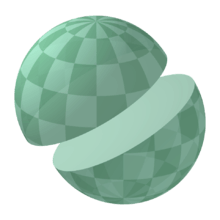Great circle on a sphere
Main article: Spherical geometry

The basic elements of Euclidean plane geometry are points and lines. On the sphere, points are defined in the usual sense. The analogue of the "line" is the geodesic, which is a great circle; the defining characteristic of the latter is that the plane containing all its points also passes through the center of the sphere. Measuring by arc length shows that the shortest path between two points lying entirely on the sphere is a segment of the great circle that includes the points.

Many theorems from classical geometry hold true for spherical geometry as well, but not all do because the sphere fails to satisfy some of classical geometry's postulates, including the parallel postulate. In spherical trigonometry, angles are defined between great circles. Thus spherical trigonometry differs from ordinary trigonometry in many respects. For example, the sum of the interior angles of a spherical triangle exceeds 180 degrees. Also, any two similar spherical triangles are congruent.

## Eleven properties of the sphere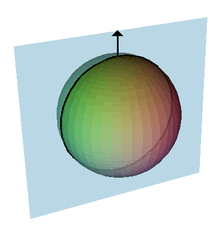A normal vector to a sphere, a normal plane and its normal section. The curvature of the curve of intersection is the sectional curvature. For the sphere each normal section through a given point will be a circle of the same radius, the radius of the sphere. This means that every point on the sphere will be an umbilical point.

In their book Geometry and the Imagination David Hilbert and Stephan Cohn-Vossen describe eleven properties of the sphere and discuss whether these properties uniquely determine the sphere. Several properties hold for the plane, which can be thought of as a sphere with infinite radius. These properties are:

1. The points on the sphere are all the same distance from a fixed point. Also, the ratio of the distance of its points from two fixed points is constant.
The first part is the usual definition of the sphere and determines it uniquely. The second part can be easily deduced and follows a similar result of Apollonius of Perga for the circle. This second part also holds for the plane.
2. The contours and plane sections of the sphere are circles.
This property defines the sphere uniquely.
3. The sphere has constant width and constant girth.
The width of a surface is the distance between pairs of parallel tangent planes. Numerous other closed convex surfaces have constant width, for example the Meissner body. The girth of a surface is the circumference of the boundary of its orthogonal projection on to a plane. Each of these properties implies the other.
4. All points of a sphere are umbilics.
At any point on a surface a normal direction is at right angles to the surface because the sphere these are the lines radiating out from the center of the sphere. The intersection of a plane that contains the normal with the surface will form a curve that is called a normal section, and the curvature of this curve is the normal curvature. For most points on most surfaces, different sections will have different curvatures; the maximum and minimum values of these are called the principal curvatures. Any closed surface will have at least four points called umbilical points. At an umbilic all the sectional curvatures are equal; in particular the principal curvatures are equal. Umbilical points can be thought of as the points where the surface is closely approximated by a sphere.
For the sphere the curvatures of all normal sections are equal, so every point is an umbilic. The sphere and plane are the only surfaces with this property.
5. The sphere does not have a surface of centers.
For a given normal section exists a circle of curvature that equals the sectional curvature, is tangent to the surface, and the center lines of which lie along on the normal line. For example, the two centers corresponding to the maximum and minimum sectional curvatures are called the focal points, and the set of all such centers forms the focal surface.
For most surfaces the focal surface forms two sheets that are each a surface and meet at umbilical points. Several cases are special:
• For channel surfaces one sheet forms a curve and the other sheet is a surface
• For cones, cylinders, tori and cyclides both sheets form curves.
• For the sphere the center of every osculating circle is at the center of the sphere and the focal surface forms a single point. This property is unique to the sphere.
6. All geodesics of the sphere are closed curves.
Geodesics are curves on a surface that give the shortest distance between two points. They are a generalization of the concept of a straight line in the plane. For the sphere the geodesics are great circles. Many other surfaces share this property.
7. Of all the solids having a given volume, the sphere is the one with the smallest surface area; of all solids having a given surface area, the sphere is the one having the greatest volume.
It follows from isoperimetric inequality. These properties define the sphere uniquely and can be seen in soap bubbles: a soap bubble will enclose a fixed volume, and surface tension minimizes its surface area for that volume. A freely floating soap bubble therefore approximates a sphere (though such external forces as gravity will slightly distort the bubble's shape).
8. The sphere has the smallest total mean curvature among all convex solids with a given surface area.
The mean curvature is the average of the two principal curvatures, which is constant because the two principal curvatures are constant at all points of the sphere.
9. The sphere has constant mean curvature.
The sphere is the only imbedded surface that lacks boundary or singularities with constant positive mean curvature. Other such immersed surfaces as minimal surfaces have constant mean curvature.
10. The sphere has constant positive Gaussian curvature.
Gaussian curvature is the product of the two principal curvatures. It is an intrinsic property that can be determined by measuring length and angles and is independent of how the surface is embedded in space. Hence, bending a surface will not alter the Gaussian curvature, and other surfaces with constant positive Gaussian curvature can be obtained by cutting a small slit in the sphere and bending it. All these other surfaces would have boundaries, and the sphere is the only surface that lacks a boundary with constant, positive Gaussian curvature. The pseudosphere is an example of a surface with constant negative Gaussian curvature.
11. The sphere is transformed into itself by a three-parameter family of rigid motions.
Rotating around any axis a unit sphere at the origin will map the sphere onto itself. Any rotation about a line through the origin can be expressed as a combination of rotations around the three-coordinate axis (see Euler angles). Therefore, a three-parameter family of rotations exists such that each rotation transforms the sphere onto itself; this family is the rotation group SO(3). The plane is the only other surface with a three-parameter family of transformations (translations along the x- and y-axes and rotations around the origin). Circular cylinders are the only surfaces with two-parameter families of rigid motions and the surfaces of revolution and helicoids are the only surfaces with a one-parameter family.

## Cubes in relation to spheres

For every sphere there are multiple cuboids that may be inscribed within the sphere. The largest cuboid which can be inscribed within a sphere is a cube.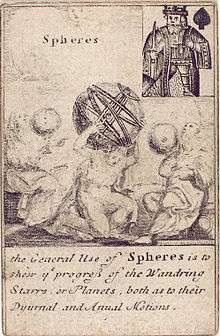Deck of playing cards illustrating engineering instruments, England, 1702. King of spades: Spheres Spherical cap Spherical coordinates Spherical Earth Spherical helix, tangent indicatrix of a curve of constant precession Spherical sector Spherical segment Spherical shell Spherical wedge Spherical zone Translation of axes Zoll sphereWikisource has the text of the 1911 Encyclopædia Britannica article Sphere.